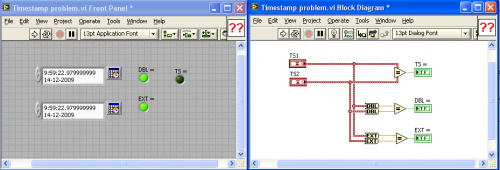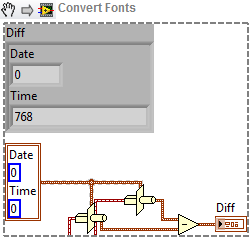# Problem comparing timestamps

## Recommended Posts

Hi,

I have two timestamps which are both calculated based on the t0 and dt of the same waveform (only diferent subvi/location).

both were attached to an in range check and i noticed the lower limit became out of range.

I copied the (equal) data from my probes to the attached vi (LV 8.5) for further testing and noticed the equal failed on the timestamp,

but converting to DBL or EXT resulted in an equal timestamp.I fixed my code by first converting to DBL before doing the compare, but I was wondering if someone has an idea what is causing this.

Timestamp problem.vi

Thanks

Hi,

I have two timestamps which are both calculated based on the t0 and dt of the same waveform (only diferent subvi/location).

both were attached to an in range check and i noticed the lower limit became out of range.

I copied the (equal) data from my probes to the attached vi (LV 8.5) for further testing and noticed the equal failed on the timestamp,

but converting to DBL or EXT resulted in an equal timestamp.I fixed my code by first converting to DBL before doing the compare, but I was wondering if someone has an idea what is causing this.

Timestamp problem.vi

Thanks

AFAIK timestamp is a 128-bit fixed precision type with 64 bits for seconds after 1.1.1904 and 64 bits for fractions of the second. My guess is that simply converting to DBL or EXT gives the same number, because for values >1 the precision of DBL and EXT is much smaller than for numbers (0..1). Try subtracting both timestamps (the result is a double) and comparing that to 0. For number <1 the precision is much higher and you should see the actual difference.

Br, Mike

Hi,

I fixed my code by first converting to DBL before doing the compare, but I was wondering if someone has an idea what is causing this.

Timestamp problem.vi

AFAIK timestamp is a 128-bit fixed precision type with 64 bits for seconds after 1.1.1904 and 64 bits for fractions of the second. My guess is that simply converting to DBL or EXT gives the same number, because for values >1 the precision of DBL and EXT is much smaller than for numbers (0..1). Try subtracting both timestamps (the result is a double) and comparing that to 0. For number <1 the precision is much higher and you should see the actual difference.

Br, Mike

The exact difference between the two timestamps is 786 out of 2^64 of a second (4,16334E-17).Ton

•1

## Join the conversation

You can post now and register later. If you have an account, sign in now to post with your account.×   Pasted as rich text.   Paste as plain text instead

Only 75 emoji are allowed.

×   Your previous content has been restored.   Clear editor

×   You cannot paste images directly. Upload or insert images from URL.

×
×